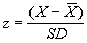Graphic of Areas Under a Normal Curve
 This graphic can be used to estimate values involving percentiles and standard scores. It can also be used to estimate confidence intervals using the standard error of measurement (SEM). Standard Scores Recall the formula for computing a standard score (z),, where X is the raw score, the X with the bar over it is the mean of the group, and SD is the standard deviation. Estimating the percentile given a standard score First, compute the standard score using the equation above. Example: Ben's score on a test having a mean of 52 and a standard deviation of 6 is 61. Using the equation, his standard score is 1.5. Next, locate (positive) 1.5 on the graphic. The point is half-way between 1SD and 2SD on the graphic. Ben's score of 61 is at the 91st percentile. There are 50% (below the mean)  + 35% (between the mean and 1SD + 7% (a little over half the percent between 1SD and 2SD). Estimating the standard score given a percentile First, locate the percentile on the graphic. Example: Matilda's score is at the 18th percentile. This point is slightly to the right of -1SD. Then estimate the standard score. (.13% + 2.15% + 13.5%) = 15.78%, or 16 percent when rounded. The area between -1SD and the mean contains 34%. Two percent takes us only slightly into the interval between -1SD and the mean. For all practical purposes, Maltida's standard score is -1 or, perhaps, -.95. Estimating confidence intervals using the SEM First, recall that the standard error of measurement (SEM) is a standard deviation. Example: The SEM for the Omnibus Test of Assessment Competencies (OTAC) is 15. Mortimer's score on the test is 40. To estimate the 68% confidence interval, take the individual's score and add and subtract 1SD in the graphic. Placing Mort's score of 40 at the mean in the graphic, we can be about 65% confident that Mort's TRUE score on the OTAC lies somewhere in the interval, 25 to 55 (i.e., between -1SD and 1SD). The 95th and 99th confidence intervals are computed in similar fashion by taking the intervals: -2SD to 2SD; and -3SD to 3SD respectfully. Similarly, we can be 95% confident that Mort's TRUE score is between 15 and 70; 99% confident that his TRUE score is between 0 and 85.